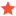• 0 Vote(s) - 0 Average
• 1
• 2
• 3
• 4
• 5
 how to calculate w,p,r angles for the robodk?DibashAdhikariMemberPosts: 20 Threads: 7 Joined: Oct 2020 Reputation: 1 04-19-2021, 07:54 AM in the example below,  https://robodk.com/doc/en/PythonAPI/exam...ram-xyzwpr there is a CSV,  actually, I also calculate the x,y,z,r,p,w and use this method, my r,p,w is wrong. because it does not give exact tool orientation.  Is there any video example available where you calculate the r,p,w values of a point. by python?  Here is the way I calculate rpw: I calculate the angle of a line (the line will give the orientation of the tool) with xy, yz, and xz planes.  the angle between XY plane and the line is the same as the angle between the line and the z-axis minus 90 degrees.  and same for the others two.  Is there any reference for calculating x,y,z,r,p,w or x,y,z,w,p,r of a point, or line in any software like a rhino. Find Reply

 Users browsing this thread: 1 Guest(s)